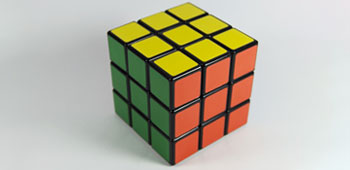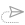## How to know or check if a number is a perfect cube?

We often need to know or check if a number is a perfect cube in order to extract the cube root of the number within a very short time, especially in the competitive examinations.

The most straight forward method is to check through factorization. If the prime factors of a number are grouped in triples of equal factors, then that number is called a perfect cube.
In order to check whether a number is a perfect cube or not, we find its prime factors and group together triplets of the prime factors. If no factor is left out then the number is a perfect cube. However if one of the prime factors is a single factor or a double factor then the number is not a perfect cube.

Examine if (i) 106480 and (ii) 19683 are perfect cubes.106480  = 2*2*2*2*5*11*11*11
One prime factor 2 and the prime factor 5 are not parts of a triplet so 106480 is not a perfect cube.

Is 19683 a perfect cube?19683 = 3*3*3*3*3*3*3*3*3

Since the prime factor 3 forms three triplets so 19683 is a perfect cube.

However, factorization method is time consuming and there is an interesting shortcut, using which we can easily and quickly check if the given number is a perfect cube or not.

Before explaining the shortcut, it is very important that the performer knows the concept of digital root or digital sum.

Let us summarize the cubes of the numbers till 10 and obtain their digital roots.

 Number Cube Digital Root of the cube 1 1 1 2 8 8 3 27 9 (or 0) 4 64 1 5 125 8 6 216 9 (or 0) 7 343 1 8 512 8 9 729 9 (or 0)

Notice, the digital root of a perfect cube is 1, 8 or 9 (0). However, the converse is not always true. That is, if a number has a digital root of 1, 8 or 9 (or 0), that does not mean, that the given number must be a perfect cube.

So, all perfect cubes must have digital root 18 or (0). Moreover, the digital root of any number's cube can be determined by the remainder the number gives when divided by 3:

• If the number is divisible by 3, its cube has digital root 9;
• If it has a remainder of 1 when divided by 3, its cube has digital root 1;
• If it has a remainder of 2 when divided by 3, its cube has digital root 8.

With this understanding, if we have to check whether a given number is a perfect cube, just obtain the digital root of the given number. If the digital root is not 1, 8 or 9 (0) we can be very sure that the number is not a perfect cube.

Consider an example. Suppose we need to check which from the following is a perfect cube:
• 91348765
• 91733851
• 91449952
• 91944321

Let us obtain the digital root of each of the given number.

The digital root of 91348765 after casting out 9′s is 7, because 9 + 1 + 3 + 4 + 8 + 7 + 6 + 5 = 43 and 4 + 3 = 7.

9 1 3 4 8 7 6 5

So, we can easily conclude 91348765 is not a perfect cube.

Now the digital root of 91733851 is 10 i.e., 1.

9 1 7 3 3 8 5 1

So, 91259809 could be a perfect cube but we cannot conclude from this.

Consider the digital root of other 2 numbers now. The digital root of 91449952 is 7.

9 1 4 4 9 9 5 2

Again we can conclude that this cannot be a perfect cube.

The digital root of 91944321 is 6.

9 1 9 4 4 3 2 1

So again, 91944321 is not a perfect cube.

So, we are left with only one option 91733851 whose digital root is 1. So, we can conclude that 91733851 is a perfect cube.

Finally to reiterate, if the digital root of a number is not 0, 1 or 8, we can easily conclude that the number is not a perfect cube. However, if a number has a digital root of 1, 8 or 0, that does not mean, that the given number must be a perfect cube.##### School reopening date: Health Ministry Issues SOP For Partial Reopening Of Schools For Students Of Classes 9th To 12th Voluntarily - Download SOP & Check Updates!

11 September, 2020, 3:36 pm7 May, 2018, 1:09 pm##### NEET 2018 Paper Analysis & Review

7 May, 2018, 11:49 am##### NEET 2018 - Dress code & Exam Pattern

4 May, 2018, 6:38 pm##### Do’s and Don'ts for NEET 2018

4 May, 2018, 1:14 pm##### JEE Advanced 2018 – Online Registration Begins

2 May, 2018, 4:30 pmWe are providing something unique, useful and most importantly fun. By giving students a tool to find instant solutions to their doubts, we’re trying to make every student self-sufficient in practicing & completing their homework

### COMPANY×